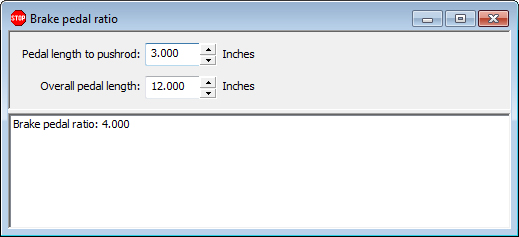## Brake Pedal Ratio Calculation

Use this calculation to determine your brake pedal ratio, which is the ratio of the amount of pressure applied by the driver's foot to the pressure exerted on the pushrod. This input is used in the brakes calculation.Enter your brake pedal length from pivot to pushrod and the overall pedal length and calculate your brake pedal ratio.

Brakes calculation

Braking force calculation

Braking time and distance calculation

Braking weight transfer calculation

Brake caliper piston area calculations

Braking graph